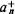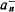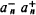# Commutation Relations

(redirected from Canonical commutation relation)
Also found in: Wikipedia.
The following article is from The Great Soviet Encyclopedia (1979). It might be outdated or ideologically biased.

## Commutation Relations

fundamental relations in quantum mechanics that establish the connection between successive operations on the wave function, or state vector, of two operators (1 and L̂2) in opposite orders, that is, between 12 and 21. The commutation relations define the algebra of the operators. If the two operators commute, that is, 12 = L̂21, then the corresponding physical quantities L1 and L2 can have simultaneously defined values. But if their commutator is nonzero, that is, L̂L̂12 – L̂21 = c, then the uncertainty principle holds between the corresponding physical quantities: ΔL̂1ΔL̂2 ≤ ǀcǀ/2, where ΔL1 and ΔL2 are the uncertainties, or dispersions, of the measured values of the physical quantities L1 and L2. The commutation relation between the operator of the generalized coordinate and its conjugate generalized momentum (q̂p̂p̂ĝ = , where ħ is Planck’s constant) is very important in quantum mechanics. If the operator commutes with the operator of the total energy of the system (the Hamiltonian) , that is, L̂Ĥ = ĤL̂, then the physical quantity L (its average value, dispersion, and so on) preserves its value in time.

The commutation relations for the operators of the creation a+ and annihilation a of particles are of fundamental importance in the quantum mechanics of systems of identical particles and in quantum field theory. For a system of free (noninteracting) bosons, the particle creation operator in the state n,and the annihilation operator for the same particle,, satisfy the commutation relationfor fermions, the relationholds. The latter commutation relation is a formal expression of the Pauli principle.

V. B. BERESTETSKII

The Great Soviet Encyclopedia, 3rd Edition (1970-1979). © 2010 The Gale Group, Inc. All rights reserved.
References in periodicals archive ?
In the so-called minimal length formulation, Kempf and Hinrichsen [16-18] have shown that the minimal length can be introduced as an additional uncertainty in position measurement, so that the usual canonical commutation relation between position and momentum operator is substituted by [x,p] = i[??](l + [beta][p.sup.2]), where [beta] is a small positive parameter called the deformation parameter.
The first is the canonical commutation relations of the infinite-dimensional Heisenberg algebra, or oscillator algebra.
They cover the structure of the space of the physical states, inequivalent representations of the canonical commutation relations, the spontaneous breakdown of symmetry and the Goldstone theorem, the dynamical rearrangement of symmetry and macroscopic manifestations of quantum field theory, thermal field theory and trajectories in the space of the representations, selected topics in thermal field theory, topological defects as non-homogeneous condensates, and dissipation and quantization.
In a very recent paper, the [sigma]-model with a two-dimensional cylindrical base space has been quantized in a new time-dependent gauge, called the cosmological gauge, that is natural to the model in the sense that the equal time commutation relations of the fields and the canonical commutation relations of their oscillating modes are compatible with each other in this gauge .
In the cosmological gauge, the canonical commutation relations of the quantum oscillators and the equal time commutation relations of the corresponding canonical fields are compatible to each other.
One can verify that the operators [X.sup.i.sub.0](t) and [[PI].sup.i.sub.0] obey the same canonical commutation relations in both representations.
In this context, the extended phase space of the particle, including time [x.sup.0] and its conjugate momentum -[p.sub.0], is quantized and canonical coordinates ([p.sub.[mu]], [x.sup.v]) become self- adjoint operators [mathematical expression not reproducible] on a kinematical Hilbert space K satisfying canonical commutation relations:
Taking the canonical commutation relations (8)-(10) and replacing (10) with (13), we obtain
This change of variables is useful because we can make [[??].sub.[mu]] and [[??].sup.v] satisfy canonical commutation relations by appropriately choosing [[SIGMA].sup.[alpha]].

Site: Follow: Share:
Open / Close